Introduction to Fixed Income Valuation | IFT World
IFT Notes for Level I CFA® Program
IFT Notes for Level I CFA® Program

# Part 5

### 3.5.     Yield Measures for Floating-Rate Notes

Floating-rate notes (FRN) are instruments where coupon/interest payments change from period to period based on a reference interest rate. Some important points to note about floating-rate notes:

• The objective is to protect the investor from volatile interest rates.
• The reference rate, usually a money market instrument such as a T-bill or an interbank offered rate like Libor, is used to calculate the interest payments. This rate is determined at the beginning of each period, but the interest is actually paid at the end of the period.
• Often, the coupon rate of an FRN is not just the reference rate, but a certain number of basis points, called the spread, is added to the reference rate.
• The specified yield spread over the reference rate is called the quoted margin.
• The spread remains constant throughout the life of the bond. The amount of spread depends on the credit quality of the issuer.

Example of a Floating Rate Note

Moody’s assigned a long-term credit rating of A2 to Nationwide, U.K.’s largest building society. Nationwide issued a perpetual floating-rate bond with a coupon rate of 6 month Libor + 240 basis points. The 2.4% quoted margin is a reflection of its credit quality. On the other hand, AAA-rated Apple sold a three-year bond at 0.05% over three-month Libor in 2013 as its credit risk was very low.

Coupon rate of a FRN = reference rate + quoted margin

The required margin is the spread demanded by the market. We saw that the quoted margin, or the spread over the reference rate, is fixed at the time of issuance. But what happens if the floater’s credit risk changes and investors demand an additional spread for bearing this risk? The required margin is the additional spread over the reference rate such that the FRN is priced at par on a rate reset date. If the required margin increases (decreases) because of a credit downgrade (upgrade), the FRN price will decrease (increase).

For example, assume a floater has a coupon rate of 3-month Libor plus 50 basis points. Six months after issuance, the issuer’s credit rating is downgraded and the market demands a required spread of 75 basis points. The coupon paid by the floater is lower than what the market demands. As a result, the floater would be priced at a discount to par as the cash flow is now discounted at a higher rate. The amount of the discount will be the present value of differential cash flows, i.e., the difference between the required and quoted margins. Conversely, if the credit rating of the issuer improves, the required margin would be below the quoted margin, and the market will demand a lower spread.

 How required margin affects a floater’s price at reset date Relationship between quoted and required margin Floater’s price at reset date Required margin = quoted margin Par Required margin > quoted margin Discount (below par) Required margin < quoted margin Premium (above par)

The required margin is also called the discount margin. FRNs can be valued using the model shown below.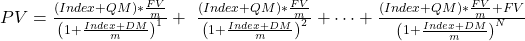where:

PV = present value of the FRN

Index = reference rate, stated as an annual percentage rate

QM = quoted margin, stated as an annual percentage rate

FV = future value paid at maturity, or the par value of the bond

m = periodicity of the floating-rate note, or the number of payment periods per year

DM = discount margin; the required margin stated as an annual percentage rate

N = number of evenly spaced periods to maturity

Equation 1 for reference: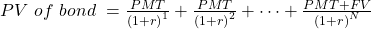How to interpret the floating-rate note equation:

• Think of it as an extension of Equation 1, we will draw similarities between the two equations.
•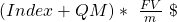is the first interest payment similar to PMT of Equation 1, which is the coupon payment per period.
• (Index + QM) is the annual rate.
• Since it is divided by periodicity we get the interest payment for that period.
• In Equation 1, cash flows are discounted at 1 + r. For FRN, the cash flow for the first period is discounted at 1+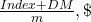for the second period at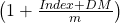, and so on.

This is considered a simple model because of the following assumptions:

• The value is calculated only on reset dates. There is no accrued interest, so the flat price is the full price.
• The model uses a 30/360 day-count convention, which means periodicity is always an integer.
• The same reference rate is used in the numerator and denominator for all payment periods.

Example

A 3-year Italian floating-rate note pays 3-month Euribor plus 0.75%. Assuming that the floater is priced at 99, calculate the discount margin for the floater if the 3-month Euribor is constant at 1% (assume 30/360 day-count convention).

Solution:

The interest payment for each period is (1.00% + 0.75%) / 4 = 0.4375%.

The keystrokes to calculate the market discount rate are: PV = -99, PMT = 0.4375, FV = 100, N = 12, CPT I/Y = 0.5237 * 4, I/Y = 2.09%

The discount margin for the floater is 2.09% – 1% = 1.09% or 109 bps.

### 3.6.     Yield Measures for Money Market Instruments

Money market instruments are short-term debt securities. They have maturities of one year or less, ranging from overnight repos to one-year certificates of deposit.

 Differences in Bond Market and Money Market Yields Bond Market Yields Money Market Yields YTM is annualized and compounded. Rate of return is annualized but not compounded; stated on a simple interest basis. YTM calculated using the standard time value of money approach using a financial calculator. Non-standard calculation using discount rates and add-on rates. YTM stated for a common periodicity for all times to maturity. Instruments with different times to maturity have different periodicities for the annual rate.

The calculation of interest of a money market instrument is different from calculating accrued interest on a bond. Money market instruments can be classified into two categories based on how the rates are quoted:

• Discount rates: T-bills, commercial paper (CP), and banker’s acceptances are discount instruments. It means they are issued at a discounted price, and pay par value at maturity. They do not make any payments before maturity. The difference between the purchase price and par value at redemption is the interest earned. Note: Do not confuse this discount rate with the rate used in TVM calculations.
Price of a money market instrument quoted on a discount basisPV = FV x (1-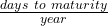x DR)
where:
PV = present value of the money market instrument
FV = face value of the money market instrument
days to maturity = actual number of days between settlement and maturity
year = number of days in the year. Most markets use a 360-day year.
DR = discount rate, stated as an annual percentage rate (APR)
Money market discount rate
Money market discount rate DR =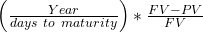FV-PV = interest earned on the instrument (this is the discount)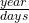= periodicity of the instrument
• Add-on rates: Bank term deposits, repos, certificates of deposit, and indices such as Libor/Euribor are quoted on an add-on basis. For a money market instrument quoted using an add-on rate, interest is added to the principal to calculate the redemption amount at maturity. In simple terms, if PV is the initial principal amount, days is the days to maturity, and year is the number of days in a year, then the amount to be paid at maturity is: FV = PV + PV x AOR xwhere AOR is the add-on rate stated on an annualized basis.
Present value or price of a money market instrument quoted on an add-on basisPV =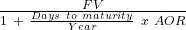where:
PV = present value of the money market instrument
FV = amount paid at maturity including interest
days = number of days between settlement and maturity
year = number of days in a year
AOR = add-on rate stated as an annual percentage rate
AOR =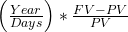Instructor’s Note

The primary difference between a discount rate (DR) and an add-on rate (AOR) is that the interest is included on the face value of the instrument for DR whereas it is added to the principal in case of AOR.

Example

Suppose that a banker’s acceptance will be paid in 91 days. It has a face value of $1,000,000. It is quoted at a discount rate of 5%. What is the price of the banker’s acceptance? Solution: PV = FV *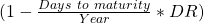FV = 1,000,000 Days = 91 Year = 360 days DR = 5% PV =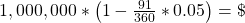987,361 Suppose that a Canadian pension fund buys a 180-day banker’s acceptance (BA) with a quoted add-on rate of 4.38% for a 365-day year. If the initial principal amount is CAD 10 million, what is the redemption amount due at maturity? Solution: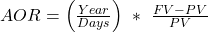0.0438 =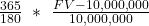FV =$10,216,000

Comparing Discount Basis with Add-On Yield

There are two approaches to compare the return of two money market instruments if one is quoted on a discount basis and the other on an add-on basis.

First approach: If you don’t want to memorize one more formula, follow this approach:

1. Determine the present value of the instrument quoted on a discount basis.
2. Use the present value to determine the AOR.
3. Compare the two AORs to see which instrument offers a better return.

Second approach: Use the following relationship between AOR and DR:

Relationship between AOR and DR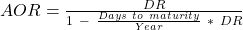Example

A T-bill with a maturity of 90 days is quoted at a discount rate of 5.25%. Its par value is \$100. Calculate the add-on rate.

Solution:

Using the first approach:

FV = 100; Days = 90; Year = 360 days; DR = 5.25%

PV =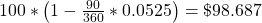AOR =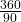*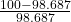= 5.32%.

Using the second approach:

AOR =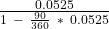= 5.3198%

Example

 Money market Instrument Quotation Basis Number of Days in the Year Quoted Rate A Discount Rate 360 3.23% B Discount Rate 365 3.46% C Add-on Rate 360 3.25% D Add-on Rate 365 3.35%

Given the four 90-day money market instruments, calculate the bond equivalent yield for each of them. Which instrument offers the highest rate of return if the credit risk is the same?

Solution:

A. The price of instrument A is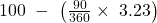= 99.1925 per 100 of par value. The bond equivalent yield is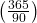×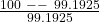= 3.30%.
B. The price of instrument B is 100 –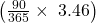= 99.1468 per 100 of par value. The bond equivalent yield is×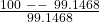= 3.49%.
C. The redemption amount per 100 of principal is 100 +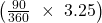= 100.8125. The bond equivalent yield is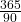× (100.8125 – 100) = 3.295%.
D. The quoted rate for instrument D of 3.35% is the bond equivalent yield. Instrument B offers the highest rate of return on a bond equivalent yield basis.

Periodicity of the Annual Rate

Another difference between yield measures in the money market and the bond market is the periodicity of the annual rate. Because bond yields-to-maturity are computed using interest rate compounding, there is a well-defined periodicity. For instance, bond yields-to-maturity for semi-annual compounding are annualized for a periodicity of two. Money market rates are computed using simple interest without compounding. In the money market, the periodicity is the number of days in the year divided by the number of days to maturity. Therefore, money market rates for different times-to-maturity have different periodicities.

Periodicity of a money market instrument =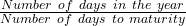Example

A 90-day T-bill has a BEY of 11%. Calculate its semiannual bond yield.

Solution:

The 11% BEY of the T-bill is based on a periodicity of 365/90. The periodicity of a semiannual bond is 2.  Give this information, we can create the equation shown below and solve for r. We will get r = 0.1115 = 11.15%.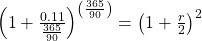** IFT Website Un-available on Monday 2-October-2023, 0700-1200 GMT **
This is default text for notification bar